ISEE Upper Level Math : Variables and Exponents

Example Questions

1 2 3 4 5 6 8 Next →

Example Question #8 : How To Divide Exponential Variables

Simplify: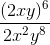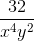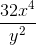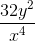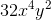Explanation: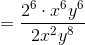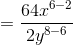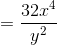Example Question #9 : How To Divide Exponential Variables

Divide the following: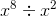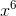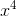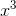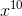Explanation:

To divide variables with exponents, we will use the following formula: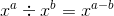Now, let’s combine the following: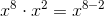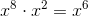Example Question #10 : How To Divide Exponential Variables

Divide the following: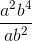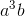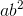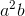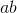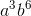Explanation:

When dividing variables, we can only divide like variable.

Also, when dividing variables with exponents, we subtract the exponents.  In other words: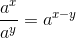So, we get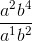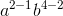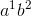Example Question #71 : Variables And Exponents

Simplify: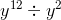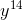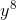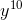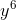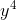Explanation:

To simplify, we will use the following formula:So, given the problem, we get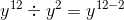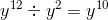Example Question #72 : Variables And Exponents

Divide the following: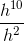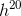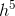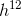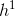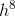Explanation:

To divide like terms with exponents, we will use the following formula:So, we get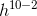Example Question #73 : Variables And Exponents

Divide the following: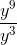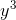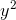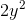Explanation:

To divide like variables with exponents, we will use the following formula:So, we get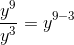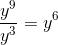Example Question #74 : Variables And Exponents

Divide the following::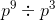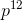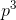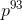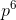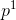Explanation:

To divide variables with exponents, we will use the following formula:Now, let’s divide the following: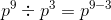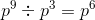Example Question #75 : Variables And Exponents

Solve the following: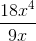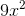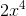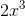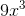Explanation:

To divide like variables with exponents, we will use the following formula:Also, we divide the coefficients like normal.

So, we get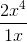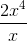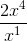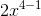1 2 3 4 5 6 8 Next →